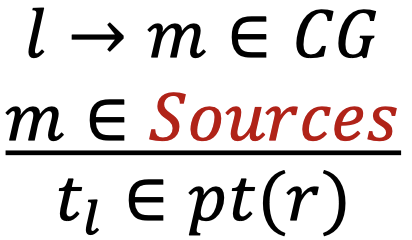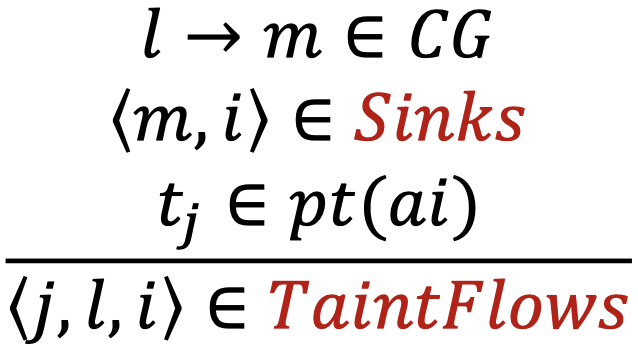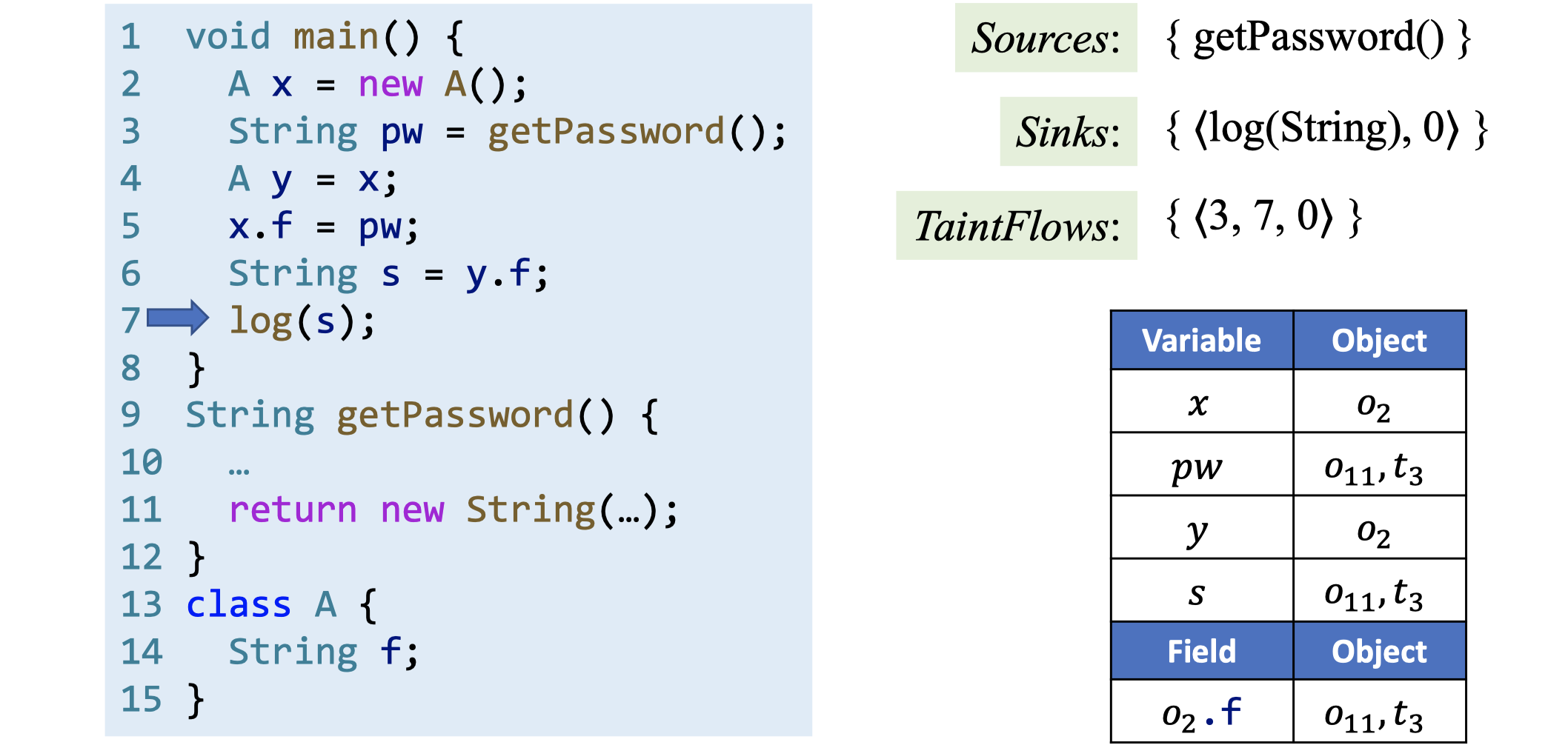## 信息流（Information Flow）安全

Dorothy E. Denning于1976年在论文A Lattice Model of Secure Information Flow提出，一个系统需要访问（access）和流（flow）控制来满足所有安全要求。

Dorothy E. Denning与Peter J. Denning夫妇二人1977年的论文Certification of Programs for Secure Information Flow对信息流做了如下解释：如果变量$x$中的信息被传送到变量$y$，它们之间就建立了一条信息流$x \rightarrow y$。这看起来与我们前面学过的指针分析十分相似。

h = getPassword(); // h is high security
broadcast(l); // l is low security


## 显式流（Explicit Flows）和隐蔽信道（Covert Channels）

secret_H = getSecret();
if (secret_H < 0) publik_L = 1;
else publik_L = 0;


• 隐式流，通过程序控制结构传递信息。
• 终止（termination）信道，通过程序的（不）可终止性差异传递信息。
• 时间（timing）信道，通过计算时间的差异传递信息。
• 异常（exceptions），通过异常来传递信息。

## 污点分析（Taint Analysis）

1. 感兴趣的数据，带有某些标签，也叫做污点数据。
2. 其他数据，或者叫无污点数据。

// information leak
y = x;
log(y); // sink

// injection error
cmd = "..." + x;
execute(cmd); // sink


Neville Grech和Yannis Smaragdakis于2017年发表的论文P/Taint: Unified Points-to and Taint Analysis指出，污点分析可以基于指针分析进行，因为两者非常相似——前者考察的是污点数据如何在程序中流动，后者考察的是抽象对象如何在程序中流动。我们只需要将污点数据当作一种特殊的“人造”对象，将sources当作污点数据的allocation sites，然后应用指针分析来传播污点数据即可。

• Variables: $x, y \in V$
• Fields: $f, g \in F$
• Objects: $o_{i}, o_{j} \in O$
• Tainted data: $t_{i}, t_{j} \in T \subset O$
• Instance fields: $o_{i}.f, o_{j}.g \in O \times F$
• Pointers: $\text{Pointer} = V \cup (O \times F)$
• Points-to relations: $pt: \text{Pointer} \rightarrow P(O)$

• Sources：由source方法（被调用后返回污点数据的方法）组成的集合。
• Sinks：由携带敏感实参的sink方法（污点数据流向这些方法的实参，违背了安全策略）组成的集合。

Call l: r = x.k(a1, ..., an)Call l: r = x.k(a1, ..., an)